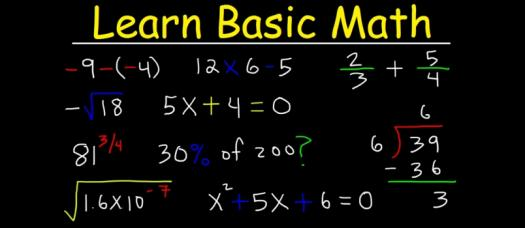# Basic Math Quiz Questions And Answers

5 Questions | Total Attempts: 1534SettingsThere is no better place to find and grow your mathematics skills that at your beginner stage. The quiz below is designed to help you practice when it comes to some basic math problems. Give it a try and keep a look out for more quizzes like this. Good luck sharpening your skills!

• 1.
What is 2 +7?
• A.

10

• B.

9

• C.

8

• D.

I have no idea

• 2.
What is 8 x 5?
• A.

13

• B.

35

• C.

40

• D.

45

• 3.
2x + 3 = 15  What is the value of x?
• A.

7

• B.

6

• C.

15

• D.

2

• 4.
X squared = 64     What is the value of x?
• A.

8

• B.

32

• C.

31

• D.

Unknown

• 5.
If x=2 then what is 4x+5 equal to?
• A.

12

• B.

10

• C.

9

• D.

13

Related Topics